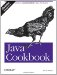# Recipe 5.1 Checking Whether a String Is a Valid Number

#### Problem

You need to check whether a given string contains a valid number, and, if so, convert it to binary (internal) form.

#### Solution

Use the appropriate wrapper class's conversion routine and catch the NumberFormat Exception. This code converts a string to a double :

`// StringToDouble.java  public static void main(String argv[]) {      String aNumber = argv;    // not argv      double result;      try {          result = Double.parseDouble(aNumber);       } catch(NumberFormatException exc) {          System.out.println("Invalid number " + aNumber);          return;      }      System.out.println("Number is " + result);  }`

#### Discussion

Of course, that lets you validate only numbers in the format that the designers of the wrapper classes expected. If you need to accept a different definition of numbers, you could use regular expressions (see Chapter 4) to make the determination.

There may also be times when you want to tell if a given number is an integer number or a floating-point number. One way is to check for the characters ., d, e, or f in the input; if one of these characters is present, convert the number as a double. Otherwise, convert it as an int:

`//  Part of GetNumber.java  private static Number NAN = new Double(Double.NaN); /* Process one String, returning it as a Number subclass  */ public Number process(String s) {     if (s.matches(".*[.dDeEfF]")) {         try {             double dValue = Double.parseDouble(s);             System.out.println("It's a double: " + dValue);             return new Double(dValue);         } catch (NumberFormatException e) {             System.out.println("Invalid a double: " + s);             return NAN;         }     } else // did not contain . d e or f, so try as int.         try {             int iValue = Integer.parseInt(s);             System.out.println("It's an int: " + iValue);             return new Integer(iValue);         } catch (NumberFormatException e2) {             System.out.println("Not a number:" + s);             return NAN;         } }`

A more involved form of parsing is offered by the DecimalFormat class, discussed in Recipe 5.8.

JDK 1.5 also features the Scanner class; see Recipe 10.5.Java Cookbook, Second Edition
ISBN: 0596007019
EAN: 2147483647
Year: 2003
Pages: 409
Authors: Ian F Darwin

Similar book on Amazon